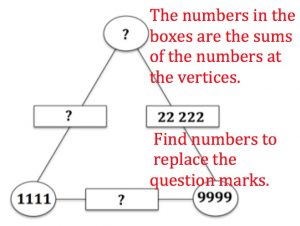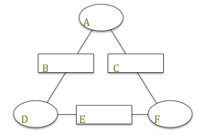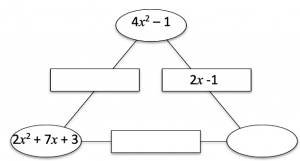Checkit can be played by 2 players or 2 teams. Each player starts with a blank Checkit frame and invents a challenge for the opponent to complete. The entries in the boxes can be any type of numbers, algebraic expressions or even words.The entries in the rectangular boxes are constructed using a rule for combining the entries at the vertices. The types of entries allowed, the rules for combining them and time allowed to complete the challenge are agreed in advance. Here are some examples:In the exponents example the numbers at the vertices are written as products of their prime factors and the numbers in the boxes are the greatest common divisors of the two numbers on that edge at the vertices.

In the algebra example the rule is that the expression in the rectangle is the common factor of the two expressions at the vertices. You could equally well make up algebraic examples for adding, subtracting, multiplying or dividing algebraic expressions including algebraic fractions.